# 农业传感网络数据差错分析算法研究Research on Data Error Analysis Algorithm of Agricultural Sensor Network

DOI: 10.12677/CSA.2018.812213, PDF, HTML, XML, 下载: 561  浏览: 3,593

Abstract: The accuracy of the agrometeorological observation elements has an important impact on the observation effect, especially in the complex environment of the field, especially the factors affecting the temperature change. At present, a large number of devices for field temperature observation are mostly wireless sensor networks with scattered nodes. The sensors used are low-cost, low-precision industrial sensors. Once they are in error, they are difficult to check and have a great impact on subsequent analysis. In order to mitigate the difficulty in searching the dysfunctional wireless sensors and targeting the problematic data, we analyze error from the perspective of agricultural sensor network data, and subsequently check out the errors in the wild wireless sensor network. By fully extracting and analyzing the standard data of the automatic station, it is found that the maximum temperature difference between different regions on the same day is approximately normal distribution on a one-year cycle, and the temperature data characteristics collected by the analog automatic station are collected by industrial sensors. The data were extracted and smoothed, and it is found that this property is also applicable to industrial sensors. Therefore we design a data error analysis algorithm, applied to wild industrial sensor calibration. By validating temperature, which is one of the essential factors in agricultural atmosphere observation, the result demonstrates the efficiency of the algorithm. Instead of directly manipulating on the current wire-less sensor network, the algorithm conducts dimensionless processing, followed by extraction, smoothing, and comparison to inspect error, to save the cost of error inspection.

1. 前言

2. 我国农业气象观测现状

3. 无线传感网络与观测要素的地域特征

3.1. 观测要素验证的确定

3.2. 温度的地域特征挖掘

${D}_{{A}_{n}{B}_{n}}={T}_{{A}_{n}}-{T}_{{B}_{n}}$ (1)

${\Psi }_{a,\tau }\left(t\right)=\frac{1}{\sqrt{a}}\Psi \left(\frac{t-\tau }{a}\right)$ (2)

${\Psi }_{a,\tau }\left(t\right)={a}_{0}^{\frac{-j}{2}}\Psi \left[{a}_{0}^{-j}\left(t-\tau \right)\right],\text{\hspace{0.17em}}\text{\hspace{0.17em}}j=0,1,2,\cdots$ (3)

$\tau =k{a}_{0}^{j}{\tau }_{0}$ (4)

${\text{WT}}_{f}\left({a}_{0}^{j},k{\tau }_{0}\right)=\int f\left(t\right){\Psi }_{{a}_{0}^{j},k{\tau }_{0}}^{*}\left(t\right)\text{d}t,\text{\hspace{0.17em}}\text{\hspace{0.17em}}j=0,1,2,\cdots ,\text{\hspace{0.17em}}\text{\hspace{0.17em}}k\in Z$ (5)

${\Psi }_{j,k}\left(t\right)={2}^{-\frac{j}{2}}\Psi \left({2}^{-j}t-k\right)$ (6)

${\text{WT}}_{f}\left(j,k\right)=\int f\left(t\right){\Psi }_{j,k}^{*}\left(t\right)\text{d}t$ (7)

$x\left(n\right)=\frac{x\left(n-2\right)+x\left(n-1\right)+x\left(n\right)+x\left(n+1\right)+x\left(n+2\right)}{5}$ (8)

$x\left(1\right)=\frac{3x\left(1\right)+2x\left(2\right)+x\left(3\right)-x\left(4\right)}{5}$ (9)

$x\left(2\right)=\frac{4x\left(1\right)+3x\left(2\right)+2x\left(3\right)+x\left(4\right)}{10}$ (10)

$x\left(m-1\right)=\frac{x\left(m-3\right)+2x\left(m-2\right)+3x\left(m-1\right)+4x\left(m\right)}{10}$ (11)

$x\left(m\right)=\frac{-x\left(m-3\right)+x\left(m-2\right)+2x\left(m-1\right)+3x\left(m\right)}{5}$ (12)

4. 仿真结果及特性分析

4.1. 模型建立与仿真Table 1. A point daily maximum temperature tableTable 2. B point daily maximum temperature tableTable 3. Maximum temperature difference between A & B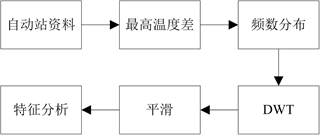Figure 1. Automatic station analysis model framework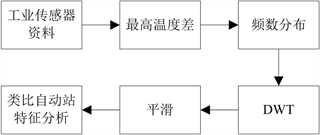Figure 2. Industrial sensor validation model framework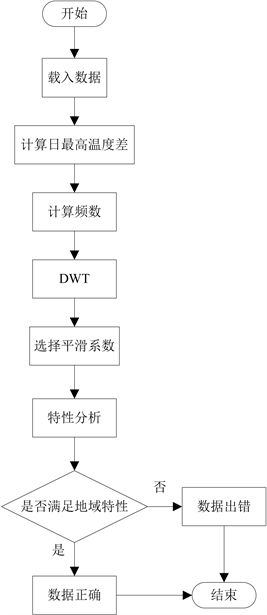Figure 3. Error detection flow chart

4.2. 特性分析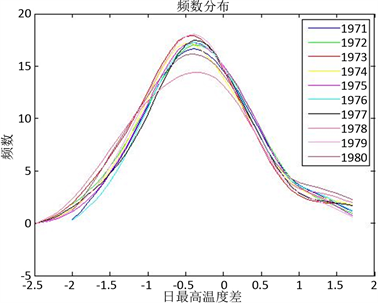Figure 4. Frequency distribution curve of the highest temperature difference between A and B

4.3. 检错可行性验证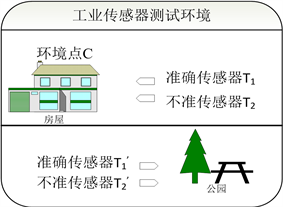Figure 5. Industrial sensor test environment diagram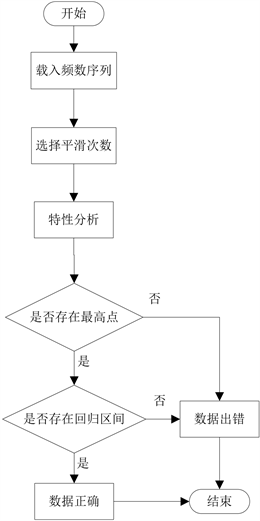Figure 6. Error detection decision flow chart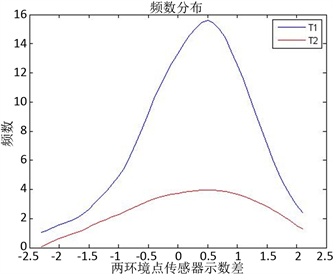Figure 7. Error detection test result chart

5. 总结与展望

1) 数据的利用：由于数据的差异型，本文只用了人工观测的数据，未来可以从自动站与人工检测的数据同步方向入手，将自动站的数据进行相应的平滑、筛选、补偿处理，将其与人工观测的数据结合起来分析。

2) 工业传感器的矫正：本文仅是提供了通过数据检测传感器好坏的方法，但对于出错数据的矫正方法仍有待研究，如可以采用游走拓扑等方式。

3) 工业传感器的精度提升：在本文提出的检测方法基础上，可以利用最高温度差的回归特性，将工业传感器向计量传感器的精度进行修正，使成本低的工业传感器经过数据修正达到计量级精度。

  谢秀英, 谢秀琴, 宁金鸽, 候向阳, 阿依奴. 农业气象观测技术要点分析[J]. 现代农业科技, 2017(12): 232+235.  陈诚, 李必军, 张永博. 基于无线传感器网络的农业信息采集系统设计[J]. 安徽农业科学, 2016, 44(10): 242-245.  Kanawaday, A., et al. (2017) Machine Learning for Predictive Maintenance of Industrial Ma-chines using IoT Sensor Data. 2017 8th IEEE International Conference on Software Engineering and Service Science (ICSESS), Beijing, 24-26 November 2017, 4. https://doi.org/10.1109/ICSESS.2017.8342870  余卫东, 杨光仙, 张志红. 我国农业气象自动化观测现状与展望[J]. 气象与环境科学, 2013, 36(2): 66-71.  蒋运志, 陈宗行, 马新建, 蒋祖林. 农业气象观测中需注意的问题[J]. 现代农业科技, 2010(15): 338.  Zhang. C.J. (2014) A Sensor Grouping Method for Industrial Sensor Health Management. Proceedings of 2014 International Conference on Future Mechanical Engineering and Materials Engineering (MEME 2014), Information Engineering Research Institute, USA, 6.  赵劲松, 李元, 邱彤. 一种基于小波变换与神经网络的传感器故障诊断方法[J]. 清华大学学报(自然科学版), 2013, 53(2): 205-209+221.  丁硕, 常晓恒, 巫庆辉, 杨友林, 胡庆功. 基于Elman神经网络的传感器故障诊断研究[J]. 国外电子测量技术, 2014, 33(4): 72-75.  姚建成. 气象对农作物生长的影响[J]. 农业与技术, 2017, 37(20): 244.  王健. 中美股市联动性——基于极大重叠离散小波变换的研究[J]. 世界经济文汇, 2014(2): 72-89.  何乃强, 惠晓滨, 周漩. 基于正态区间估计的改进型无量纲化方法[J]. 计算机工程与应用, 2012, 48(5): 236-238.  杨春庄. 改进的滑动窗平滑声能衰减曲线法估值混响时间[C]//中国声学学会. 泛在信息社会中的声学——中国声学学会2010年全国会员代表大会暨学术会议论文集. 北京: 中国声学学会, 2010: 2.  陈成国, 石慧兰, 王桂兰, 邵志勇, 周圣军. 自动与人工气象观测要素的差异及原因分析[J]. 安徽农业科学, 2010, 38(35): 20222-20224+20235.